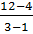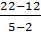### Sample Problem

Line A runs through points (1,4) and (3,12)

Line B runs through points (2,10) and (5,22)

These lines are

#### Solution

The slope of line A is= 4

The slope of line B is= 4

Because these slopes are equal to one another, we can conclude that lines A and B are parallel.